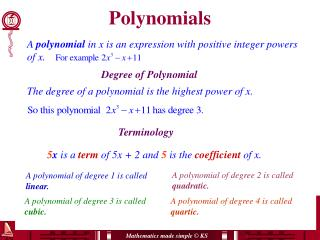# Polynomials - PowerPoint PPT PresentationDownload PresentationPolynomials

PolynomialsDownload Presentation## Polynomials

- - - - - - - - - - - - - - - - - - - - - - - - - - - E N D - - - - - - - - - - - - - - - - - - - - - - - - - - -
##### Presentation Transcript

1. Polynomials A polynomial in x is an expression with positive integer powers of x. Degree of Polynomial The degree of a polynomial is the highest power of x. Terminology 5x is a term of 5x + 2 and 5 is the coefficient of x. A polynomial of degree 2 is called quadratic. A polynomial of degree 1 is called linear. A polynomial of degree 3 is called cubic. A polynomial of degree 4 is called quartic.

2. Adding and subtracting polynomials Like terms: Like terms have identical letters and powers. Simplify (5x4– x3+ 8x) + (2x4 + 11x + 6) =7x4 – x3 + 19x +6 The usual convention is to write the polynomial with the highest power of x first. Simplify (5x3– 4xy + 8) + (2x3 + 11x + 1) = 7x3 – 4xy + 11x + 9 Simplify Take care when subtracting (3x2 + 5x – 11) – (x2 – 7x – 20) 3x2 + 5x – 11 – x2 +7x + 20 = 2x2 + 12x + 9

3. Expanding brackets Simplify 3(2x2 – 5x + 4) – 2(x2 – 3x – 1) 6x2 – 15x + 12 – 2x2 + 6x + 2 Expand brackets: Collect like terms: = 4x2 – 9x + 14 f(x) = 4x3 – 2x2 + 5x - 11 g(x) = x3 + 3x2 - 4x + 5 Simplify f(x) + 2g(x) f(x)+ 2g(x)= 4x3 – 2x2 + 5x – 11 + 2(x3 + 3x2 – 4x + 5) = 4x3 – 2x2 + 5x – 11 + 2x3 + 6x2 – 8x + 10 = 6x3 + 4x2 - 3x – 1

4. Expanding two or more brackets (a + b)(c + d) = ac + ad + bc + bd (x + 4)(x – 6) = x2 – 6x + 4x - 24 Collect like terms = x2 – 2x - 24 = a2 + 2ab + b2 (a + b)2 (a - b)2 = a2 - 2ab + b2 (a - b)(a + b) = a2 - b2 (a – b)3 = a3 - 3a2b + 3ab2 + b3 Expand two brackets then multiply by the third.

5. Three brackets Expand and simplify the polynomial (2x + 1)(x – 3)(x + 4).  Expand two of the brackets, then multiply by the third bracket

6. Identical polynomials () Find the values of a, b and c:  Expand the left hand side  Equate coefficients of corresponding powers of x  Write down the values of a, b and c a = 3, b = –1, c = 5

7. Polynomial substitution Given that P(2) = 10 , find the value of k.  Substitute x = 2 into the polynomial and equate it to 10.